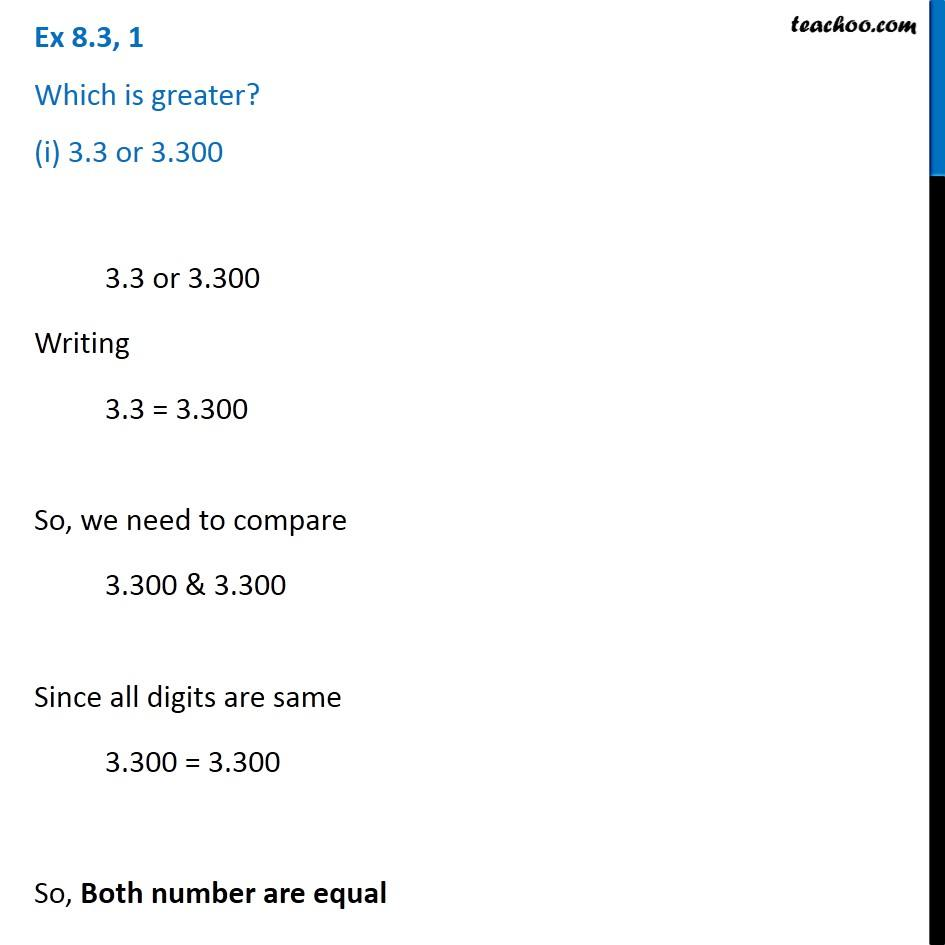1. Chapter 8 Class 6 Decimals
2. Concept wise
3. Comparing decimals

Transcript

Ex 8.3, 1 - Chapter 8 Class 6 Decimals - NCERT Book Which is greater? (i) 3.3 or 3.300 Writing 3.3 = 3.300 So, we need to compare 3.300 & 3.300 Since all digits are same 3.300 = 3.300 So, Both numbers are equal

Comparing decimals